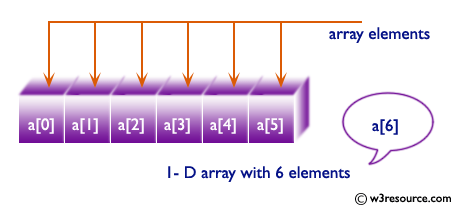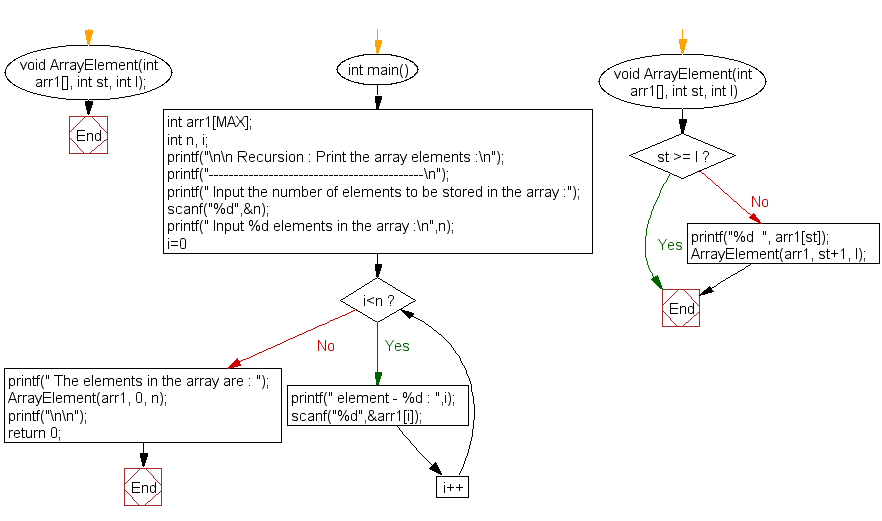﻿ C Program: Print the array elements - w3resource

# C Exercises: Print the array elements

## C Recursion : Exercise-4 with Solution

Write a program in C to print the array elements using recursion.

Pictorial Presentation:Sample Solution:

C Code:

``````#include <stdio.h>
#define MAX 100

void ArrayElement(int arr1[], int st, int l);

int main()
{
int arr1[MAX];
int n, i;
printf("\n\n Recursion : Print the array elements :\n");
printf("-------------------------------------------\n");

printf(" Input the number of elements to be stored in the array :");
scanf("%d",&n);

printf(" Input %d elements in the array :\n",n);
for(i=0;i<n;i++)
{
printf(" element - %d : ",i);
scanf("%d",&arr1[i]);
}

printf(" The elements in the array are : ");
ArrayElement(arr1, 0, n);//call the function ArrayElement
printf("\n\n");
return 0;
}

void ArrayElement(int arr1[], int st, int l)
{
if(st >= l)
return;

//Prints the current array element
printf("%d  ", arr1[st]);

/* Recursively call ArrayElement to print next element in the array */
ArrayElement(arr1, st+1, l);//calling the function  ArrayElement itself
}
```
```

Sample Output:

``` Recursion : Print the array elements :
-------------------------------------------
Input the number of elements to be stored in the array :6
Input 6 elements in the array :
element - 0 : 2
element - 1 : 4
element - 2 : 6
element - 3 : 8
element - 4 : 10
element - 5 : 12
The elements in the array are : 2  4  6  8  10  12
```

Explanation:

```void ArrayElement(int arr1[], int st, int l)
{
if(st >= l)
return;

//Prints the current array element
printf("%d  ", arr1[st]);

/* Recursively call ArrayElement to print next element in the array */
ArrayElement(arr1, st+1, l);//calling the function  ArrayElement itself
}
```

This ArrayElement() function takes an array arr1 of integers and its starting index st and ending index l as arguments. It recursively prints each element of the array starting from the element at index st up to the element at index l-1. It does this by first checking if st is greater than or equal to l, in which case it returns and stops the recursion. Otherwise, it prints the element at index st using printf() function, and then recursively calls the ‘ArrayElement’ function with the next index (st+1) as the new starting index.

Time complexity and space complexity:

The time complexity of this function is O(n), where n is the number of elements in the array.

The space complexity of the function is O(n) as well, due to the use of the function call stack to maintain the recursion. The maximum space used on the stack is equal to the maximum depth of the recursion, which is equal to n for this function.

Flowchart:C Programming Code Editor:

Have another way to solve this solution? Contribute your code (and comments) through Disqus.

What is the difficulty level of this exercise?

Test your Programming skills with w3resource's quiz.

﻿

## C Programming: Tips of the Day

__FILE__ macro shows full path

```#include <string.h>

#define __FILENAME__ (strrchr(__FILE__, '/') ? strrchr(__FILE__, '/') + 1 : __FILE__)
For Windows use '\\' instead of '/'.
```

Ref : https://bit.ly/3iEWRoT

We are closing our Disqus commenting system for some maintenanace issues. You may write to us at reach[at]yahoo[dot]com or visit us at Facebook